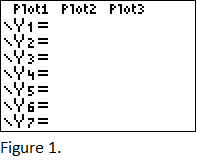# How to Evaluate & Graph Functions on a Graphing Calculator

Instructor: Miriam Snare

Miriam has taught middle- and high-school math for over 10 years and has a master's degree in Curriculum and Instruction.

This lesson will teach you how to use a graphing calculator to evaluate a function at a given value and how to graph a function. It will also give you some trouble-shooting tips for these tasks.

## Introduction

Evaluating a function and graphing a function are done on different screens of a graphing calculator. This lesson will explain how to do each of these on most Texas Instrument calculators. If you have a different brand of calculator, your screens and buttons may be different.

## Evaluating Functions

Evaluating a function can be done on the main calculator screen, the one where you do your usual number operations. Let's go through an example, and I'll explain the steps. We are going to evaluate the function f(x) = 2x^3 - 5x^2 + 4x - 7 when x = 2.

### Storing the Value of the Variable

First, we need to store the value of the variable that we want to substitute into the function, the x = 2. We do that by entering 2 and then hitting the button labeled STOR> and then entering a letter (you may need also hit ALPHA before selecting a letter). On some calculators, the x is conveniently located on the same button as STOR>. After you have input the number and the letter under which to store it, you just hit enter. The calculator will show the number, in this example 2, to confirm what you entered.

### Entering the Function

Next, you just need to enter the function, leaving off the f(x) = part. Punch in 2x^3 - 5x^2 + 4x - 7 exactly as it is written. Make sure to include the exponents correctly. Since you stored 2 as the x-value, the calculator will automatically insert 2 for every x and evaluate the function. When you hit ENTER after typing in the function, the calculator will display the correct function value of -3.

Just one more important note for evaluating functions on the calculator: Make sure you enter the value of the variable first every time you want to evaluate a new function or a new variable value. If you don't tell the calculator the new value, it will just use the last variable value that was stored. If you don't ever store a value under a particular letter, the default value is 0.

## Graphing Functions

In order to graph functions, you need to use the buttons in the row right underneath the screen. You are going to start with the first button on the left of that row, the one labeled Y=. When you hit that button, you get a screen shown in Figure 1.### Entering the Function

The cursor will be blinking in the row labeled Y1 =. You need to type in the function you want to graph. That function needs to be solved for y and contain only the variable x on the right side of the equation. If your function contains a different variable, you still need to enter x in place of that variable because the calculator does not recognize a different letter while graphing.

So, let's graph the same function we used in the previous example, f(x) = 2x^3 - 5x^2 + 4x - 7. We leave off the f(x) = part and enter 2x^3 - 5x^2 + 4x - 7 right after the Y1 =. Then you have to hit the GRAPH button on the right end of the button row under the screen.

To unlock this lesson you must be a Study.com Member.

### Register to view this lesson

Are you a student or a teacher?